PSAT Math : Tetrahedrons

Example Questions

Example Question #252 : Psat Mathematics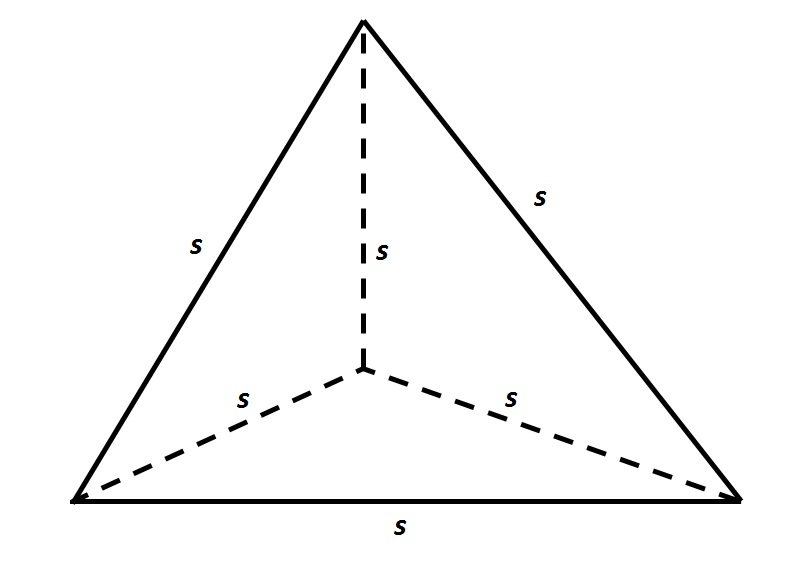Refer to the above tetrahedron, or four-faced solid. The surface area of the tetrahedron is 444. Evaluateto the nearest tenth.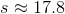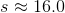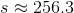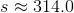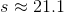Explanation:

The tetrahedron has four faces, each of which is an equilateral triangle with sidelength. Since the total surface area is 444, each triangle has area one fourth of this, or 111. To find, set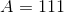in the formula for the area of an equilateral triangle: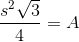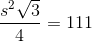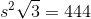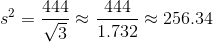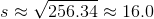Example Question #253 : Psat Mathematics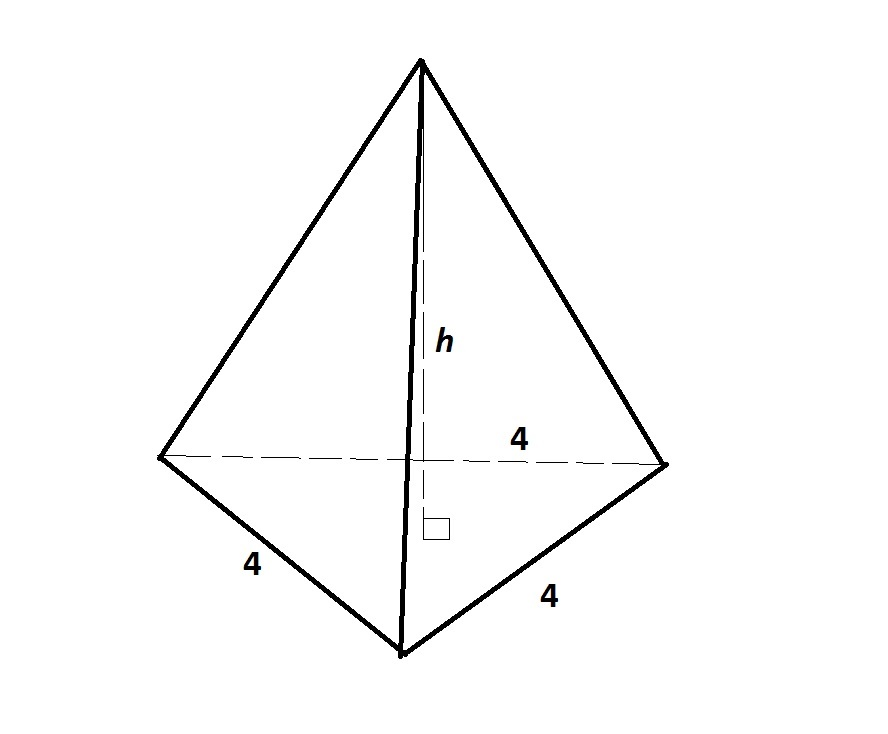Note: Figure NOT drawn to scale.

The above triangular pyramid has volume 25. To the nearest tenth, evaluate.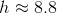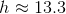Insufficient information is given to answer the problem.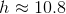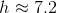Explanation:

We are looking for the height of the pyramid.

The base is an equilateral triangle with sidelength 4, so its area can be calculated as follows: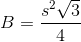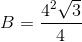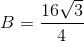The heightof a pyramid can be calculated using the fomula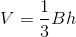We set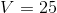and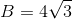and solve for: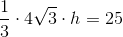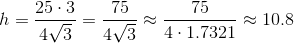Example Question #254 : Psat Mathematics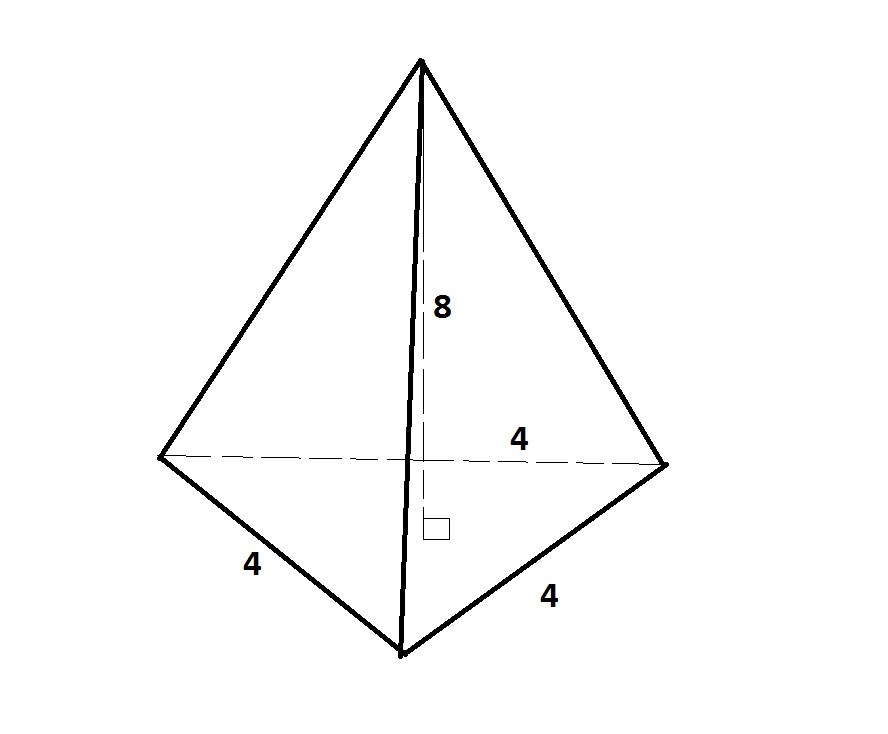Note: Figure NOT drawn to scale.

Give the volume (nearest tenth) of the above triangular pyramid.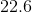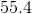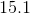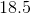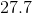Explanation:

The height of the pyramid is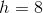. The base is an equilateral triangle with sidelength 4, so its area can be calculated as follows:The volume of a pyramid can be calculated using the fomula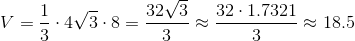Example Question #255 : Psat Mathematics

A regular tetrahedron has an edge length of. What is its volume?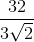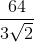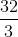Explanation:

The volume of a tetrahedron is found with the equation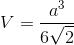, whererepresents the length of an edge of the tetrahedron.

Plug in 4 for the edge length and reduce as much as possible to find the answer: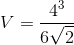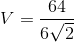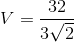The volume of the tetrahedron is.

Example Question #256 : Psat Mathematics

A regular tetrahedron has four congruent faces, each of which is an equilateral triangle.

A given tetrahedron has edges of length six inches. Give the total surface area of the tetrahedron.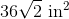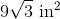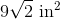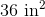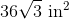Explanation:

The area of an equilateral triangle is given by the formula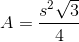Since there are four equilateral triangles that comprise the surface of the tetrahedron, the total surface area is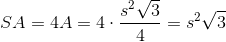Substitute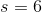: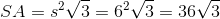square inches.

Example Question #257 : Psat Mathematics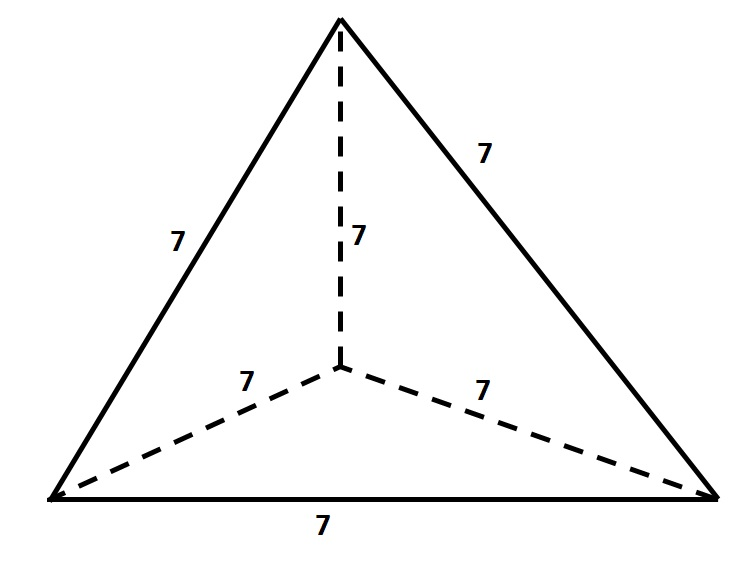Give the surface area of the above tetrahedron, or four-faced solid, to the nearest tenth.

Insufficient information is given to answer the question.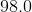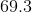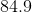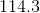Explanation:

The tetrahedron has four faces, each of which is an equilateral triangle with sidelength 7. Each face has area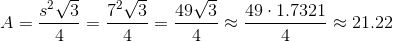The total surface area is four times this, or about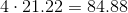.

Rounded, this is 84.9.